# NCERT Exemplar Class 8 Maths Chapter 2 - Linear equations in one variable - Free PDF download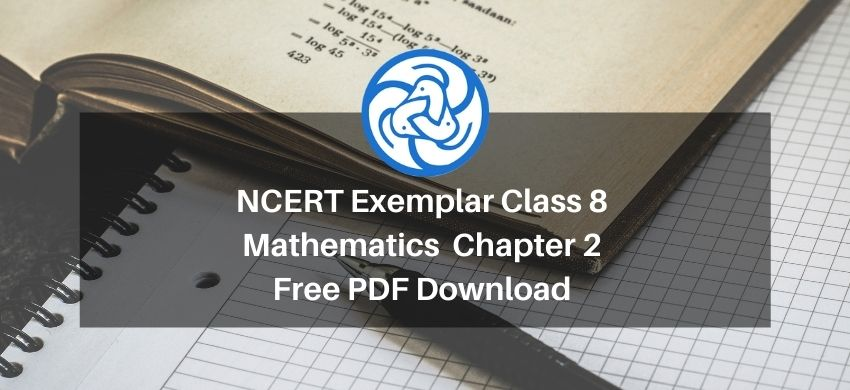Hey, are you a class 8 student? and looking for ways to download NCERT Exemplar Class 8 Maths Chapter 2 "Linear equations in one variable"? If yes. Then read this post till the end.

If you want to score high in your Class 8 Maths exam then it is very important for you to understand and learn the topics of Class 8 Maths thoroughly.

So, without wasting more time let’s start.

## Download The PDF of NCERT Exemplars Class 8 Maths Chapter 2 "Linear equations in one variable"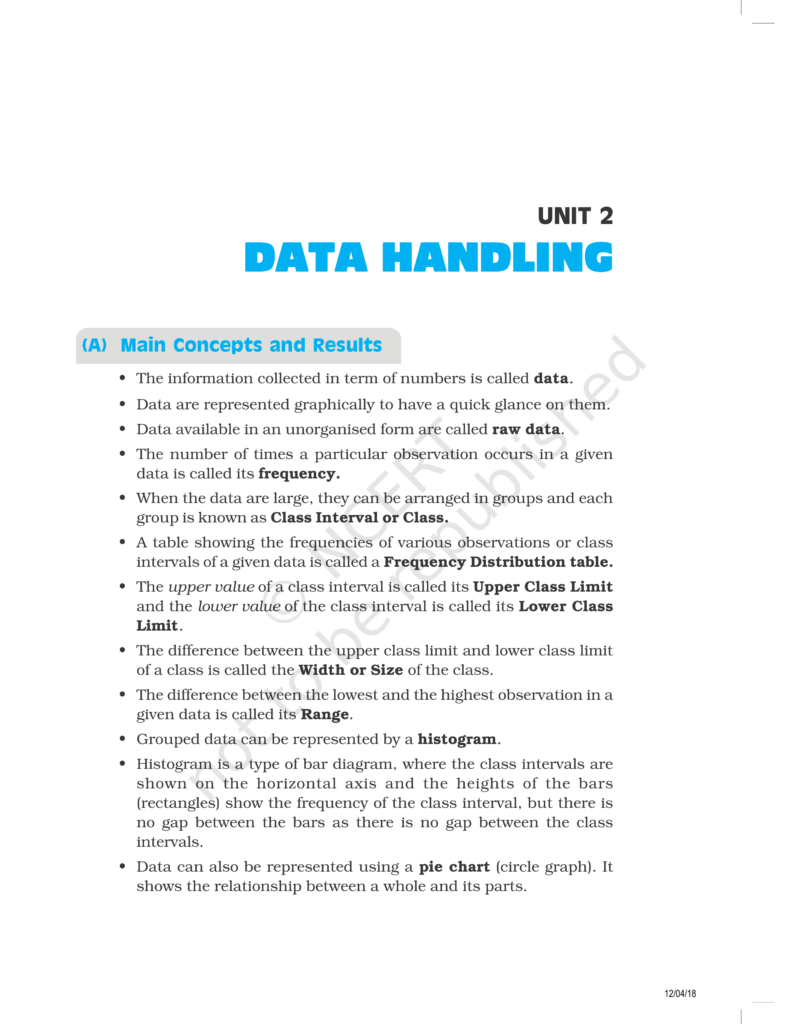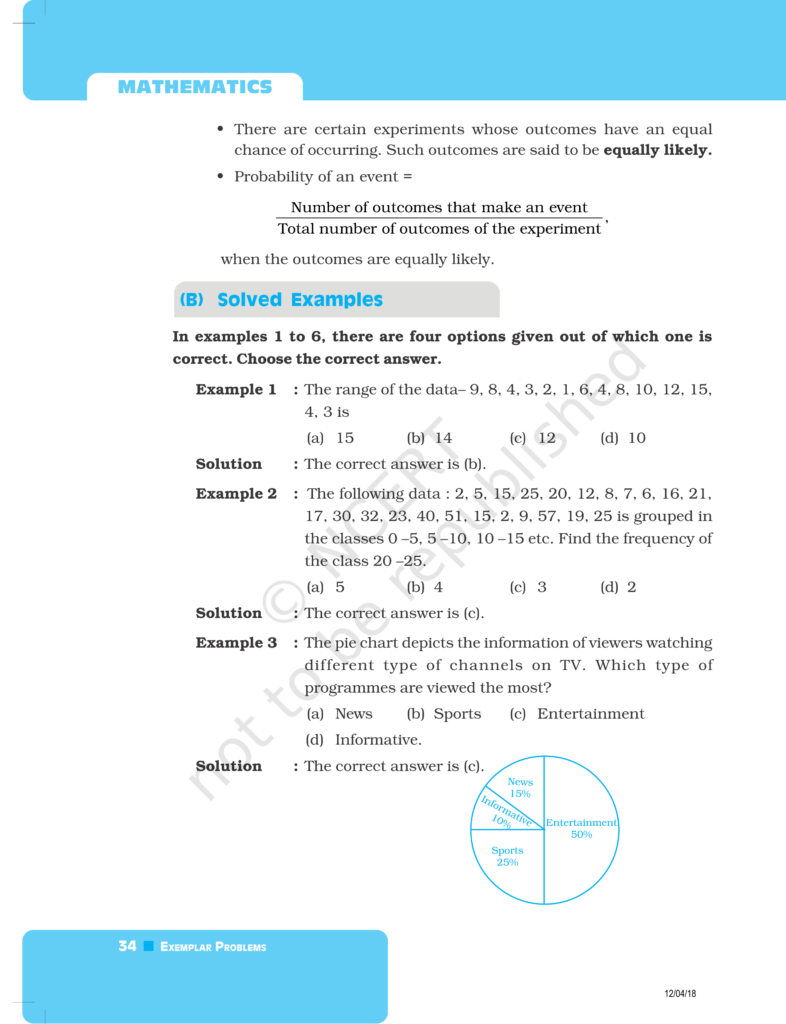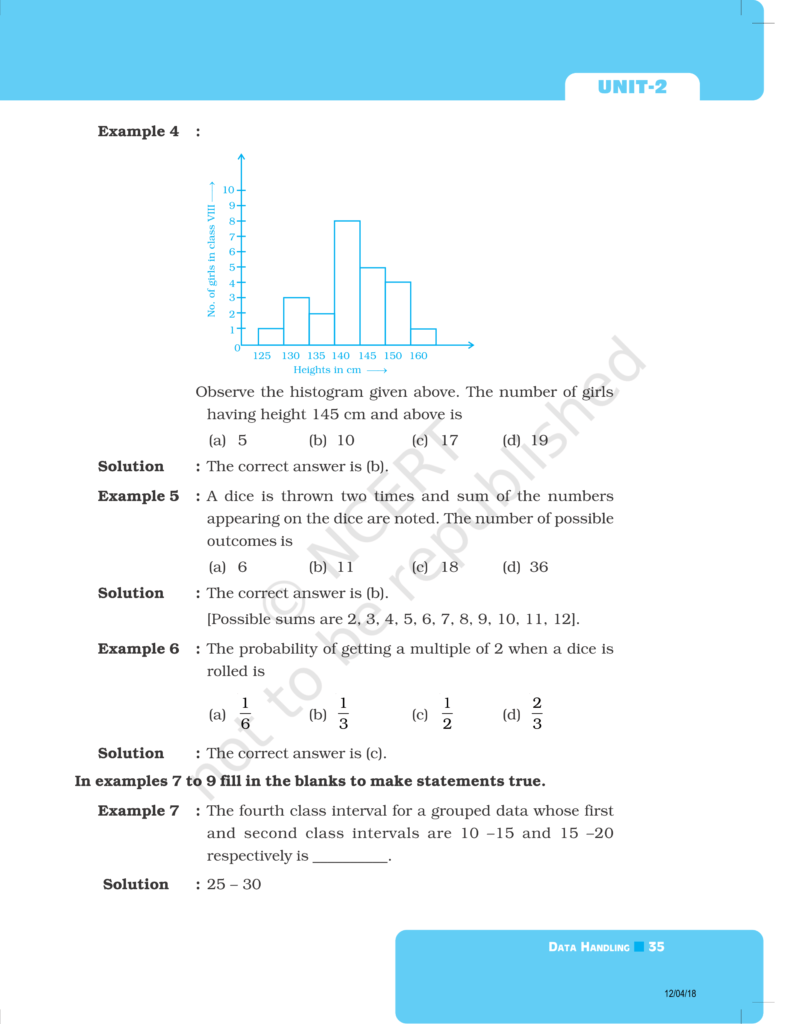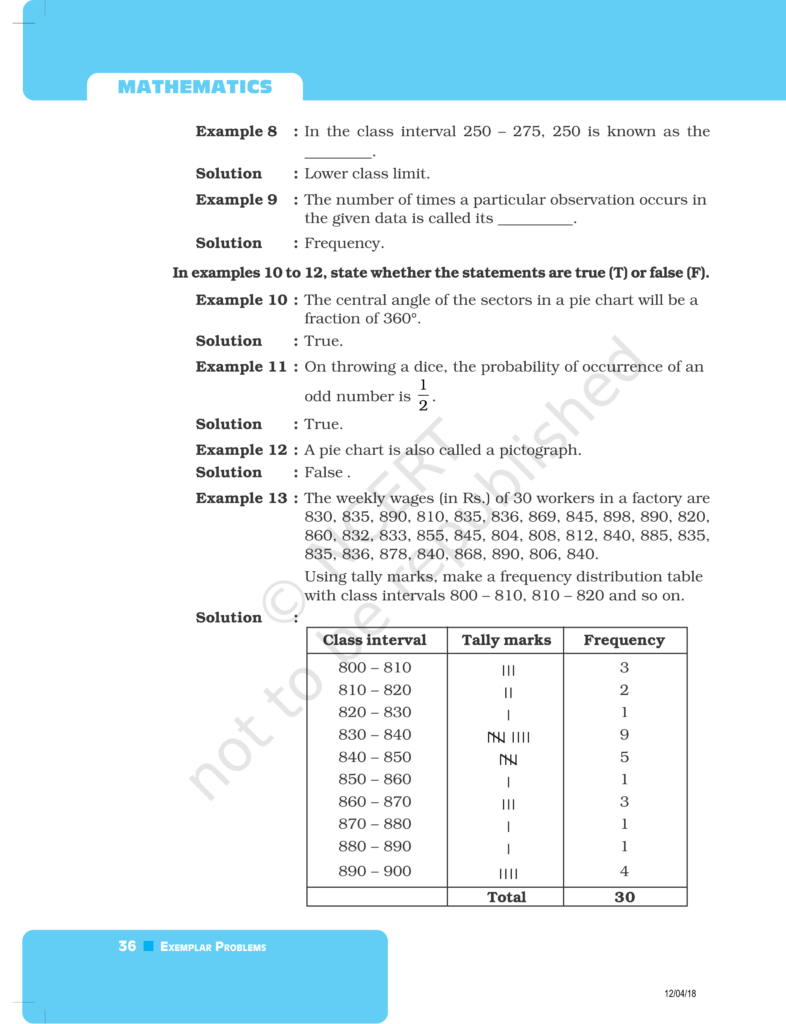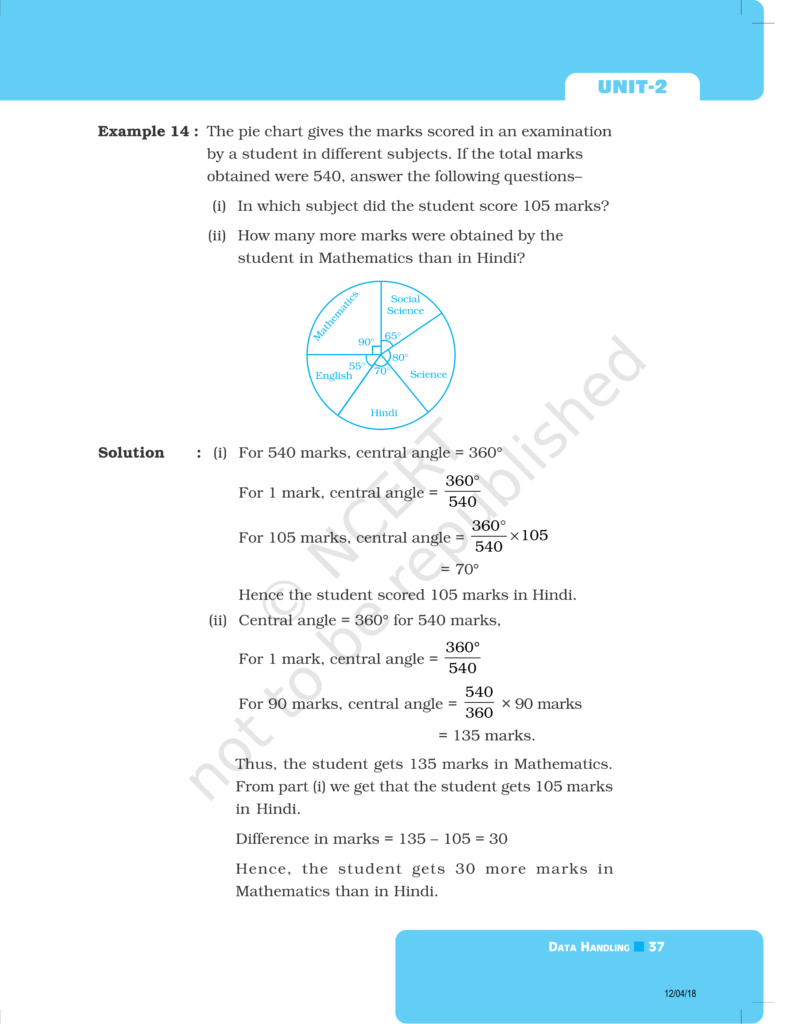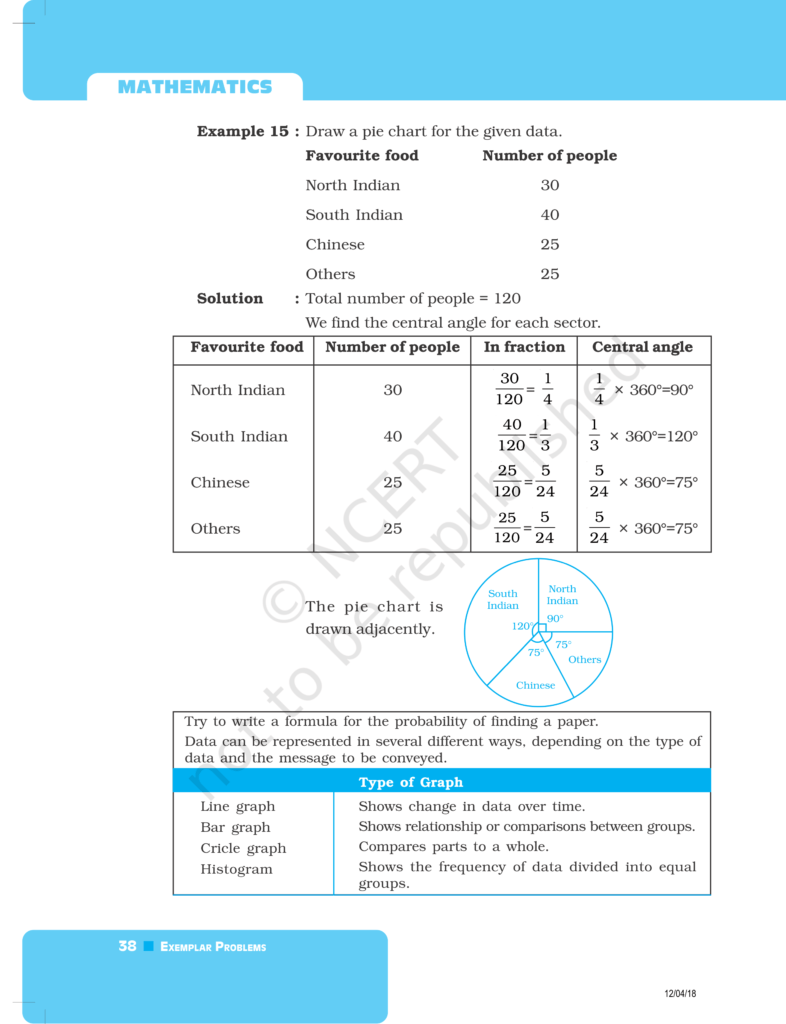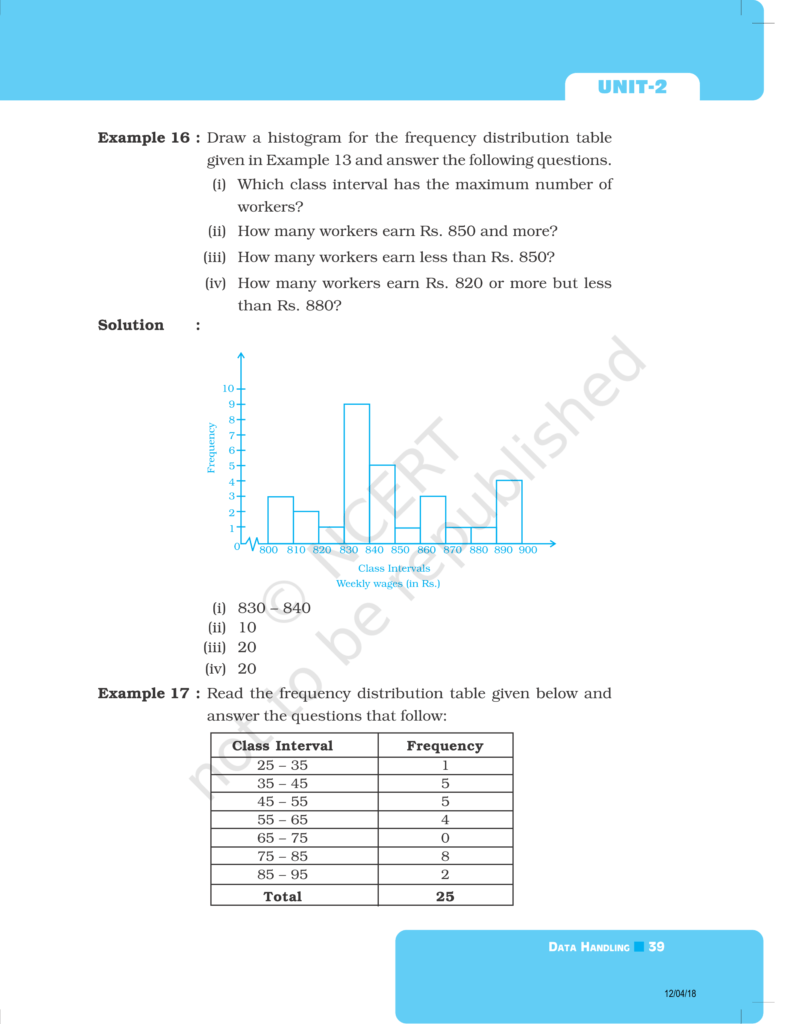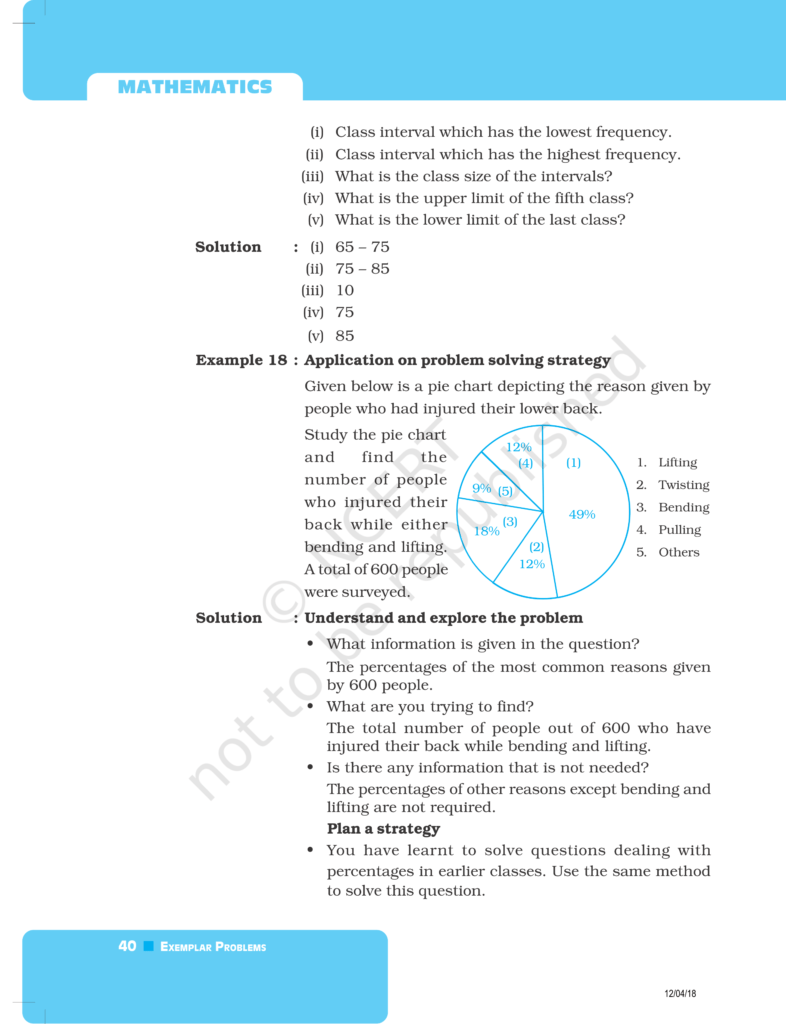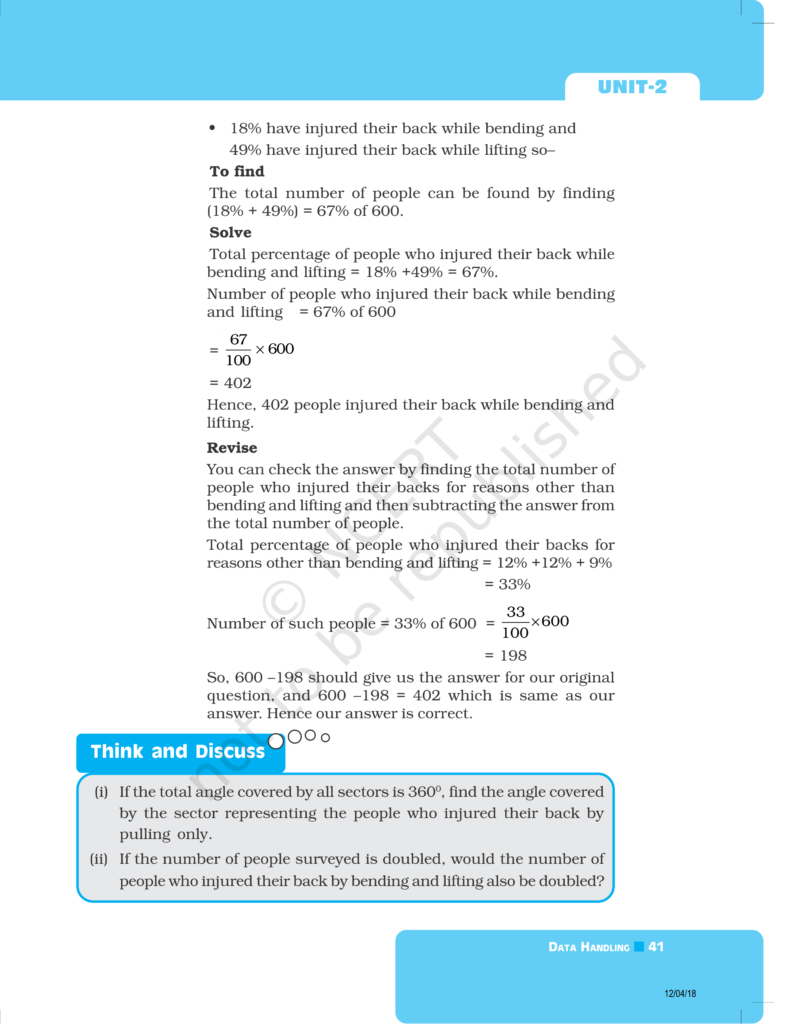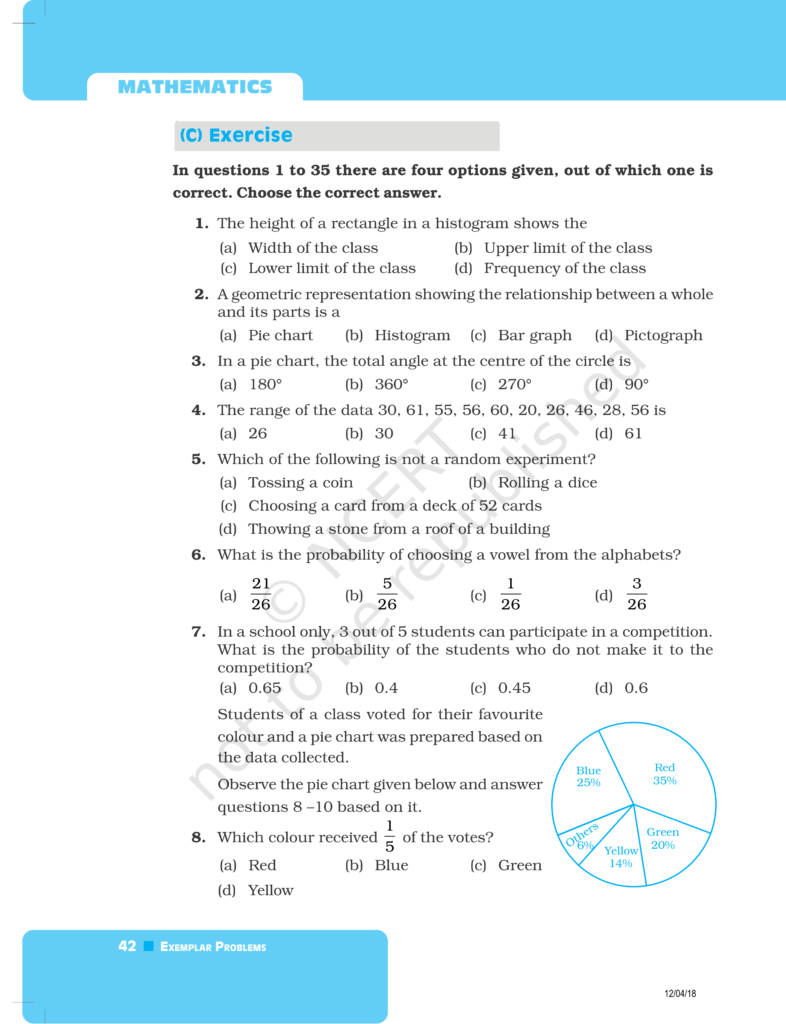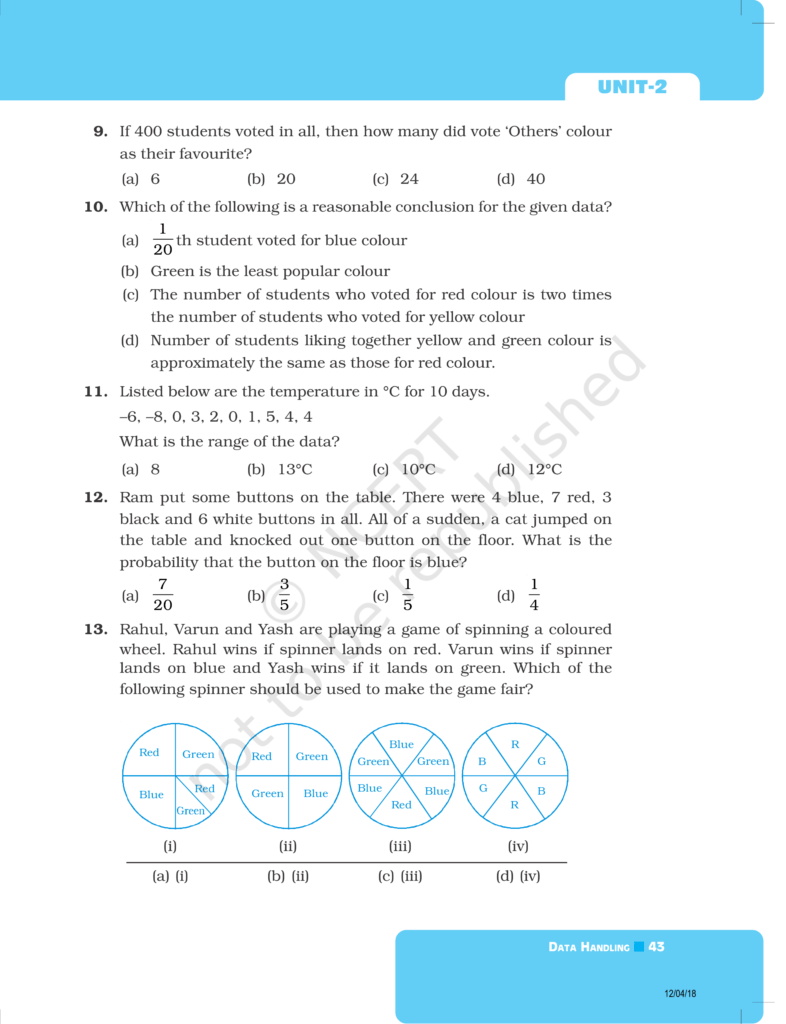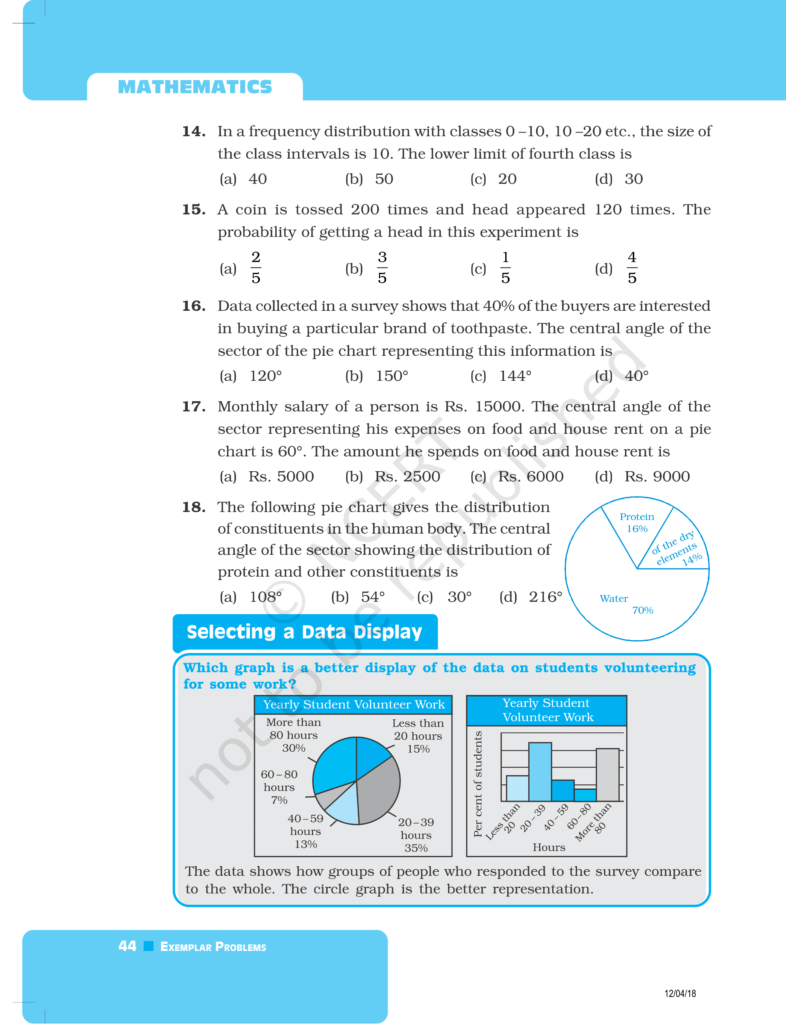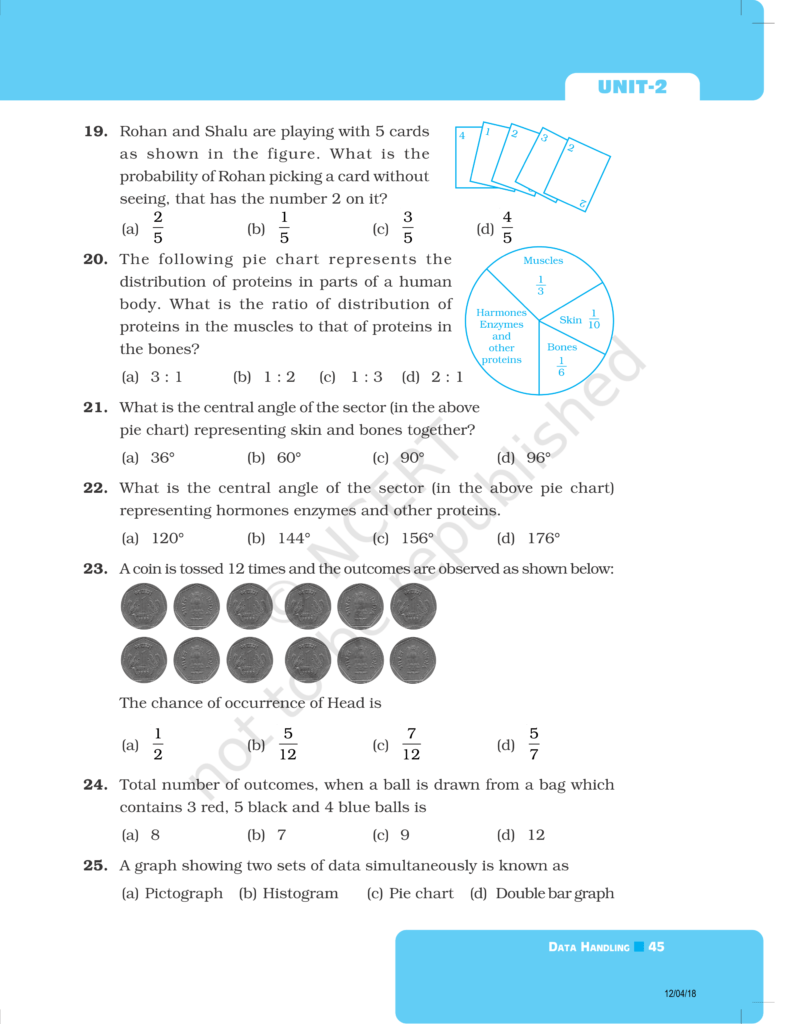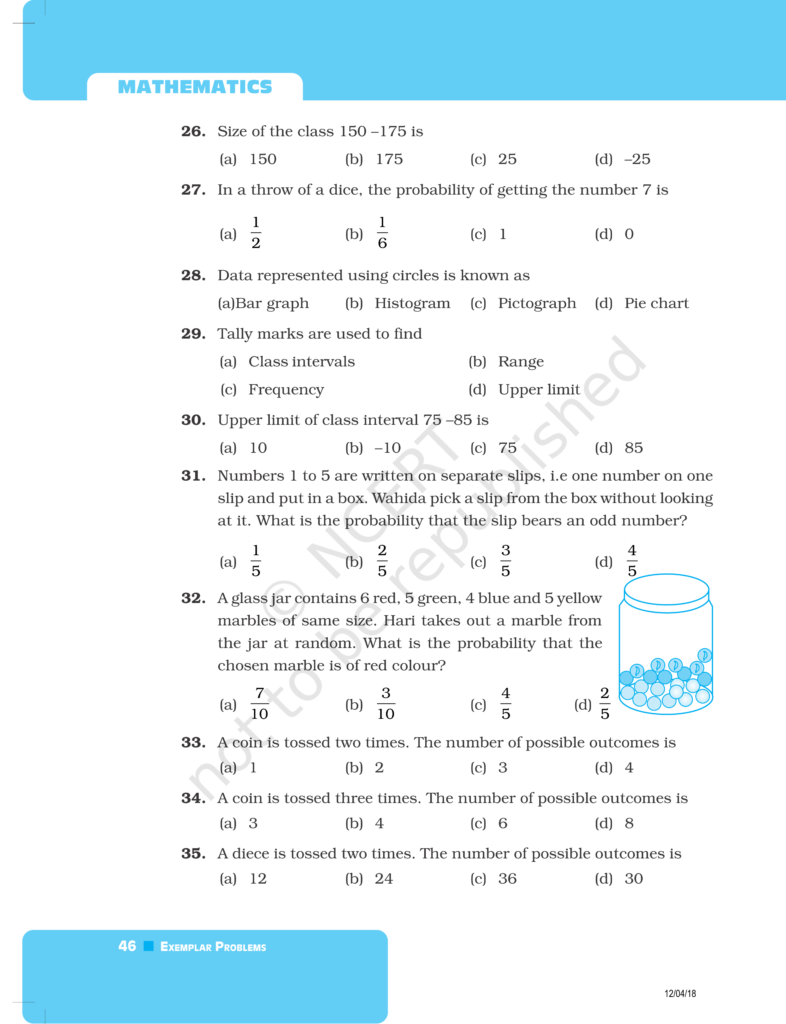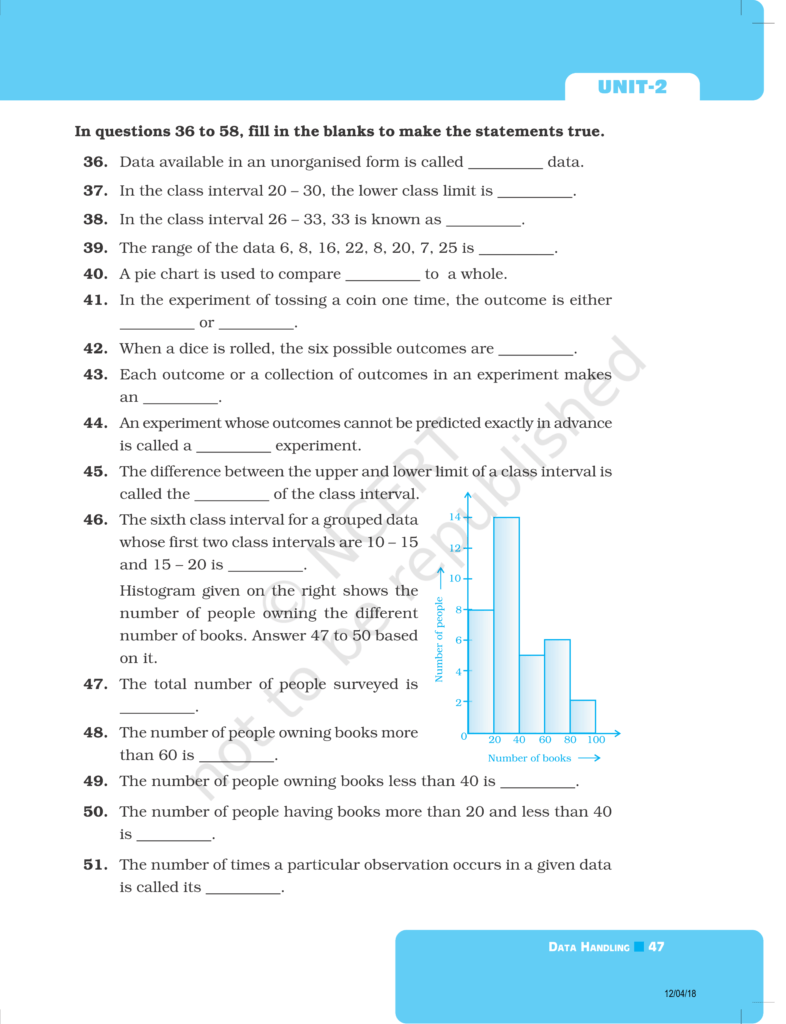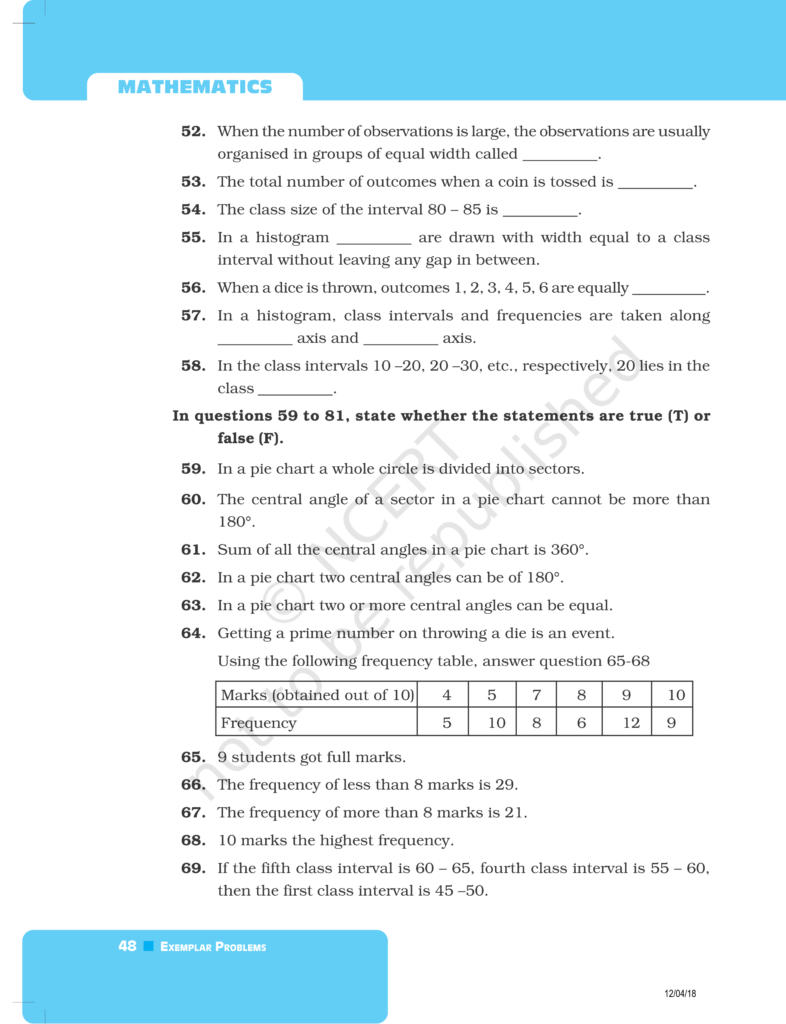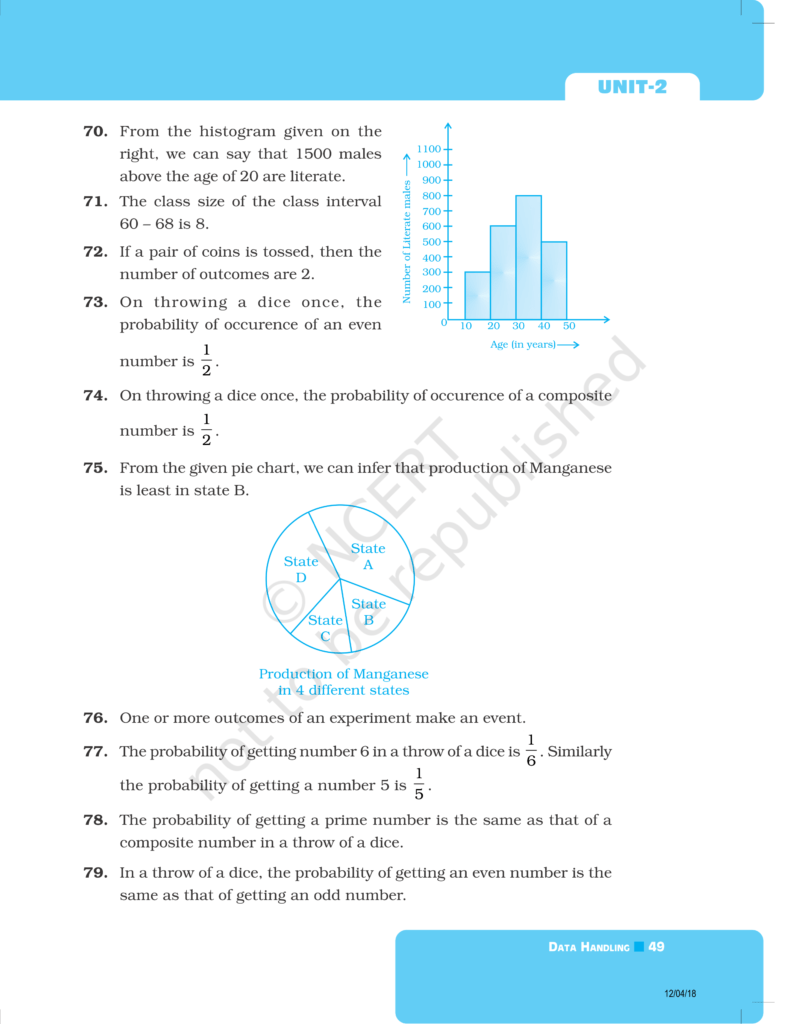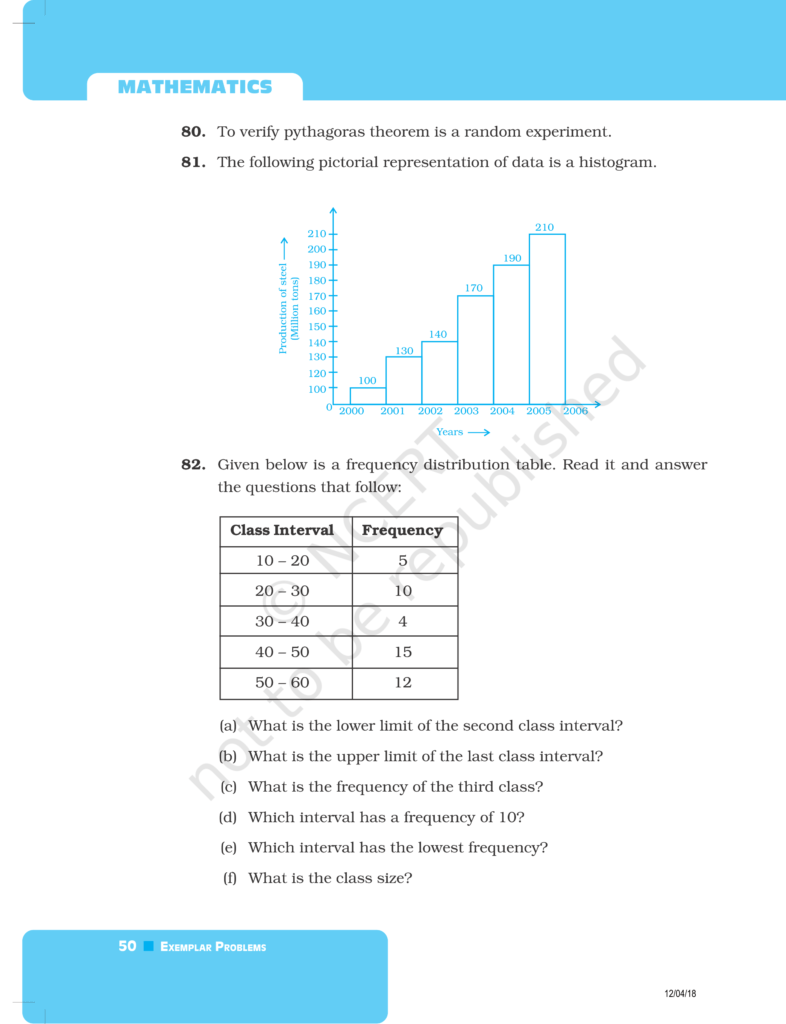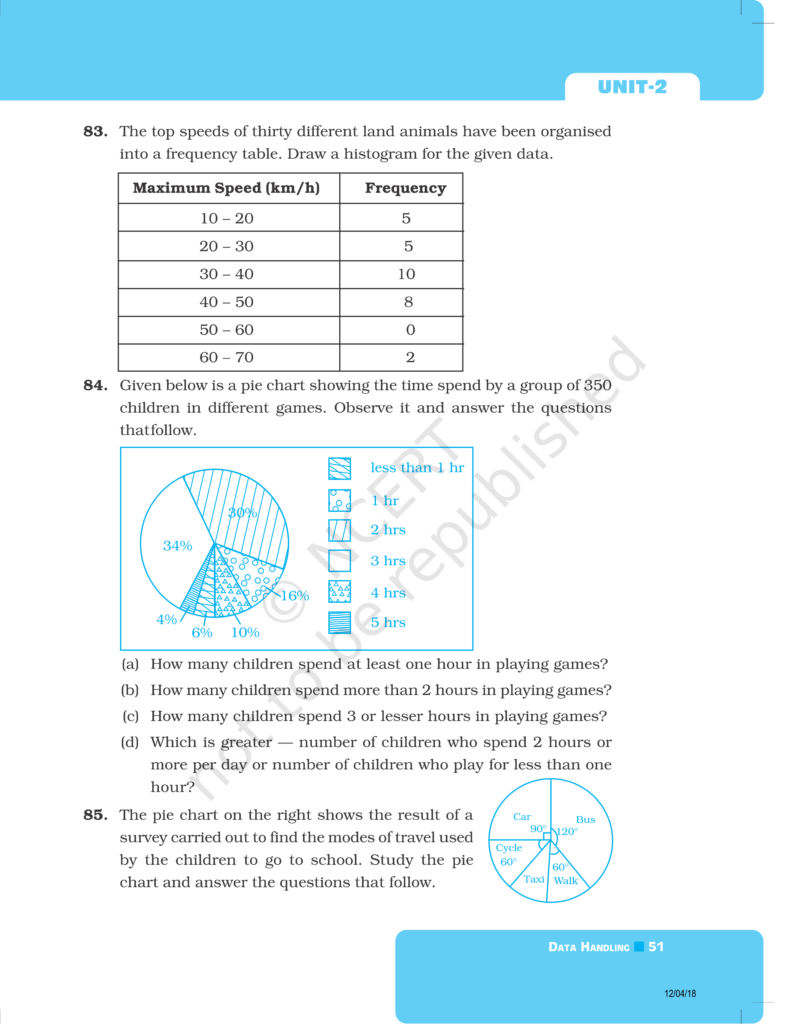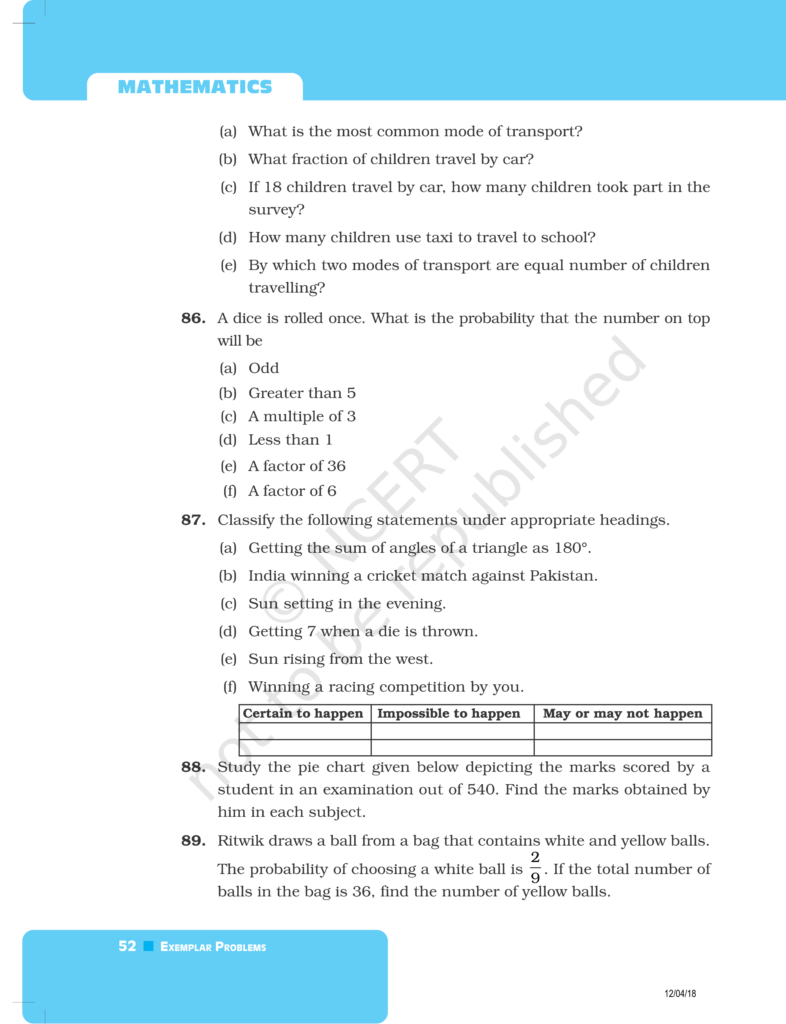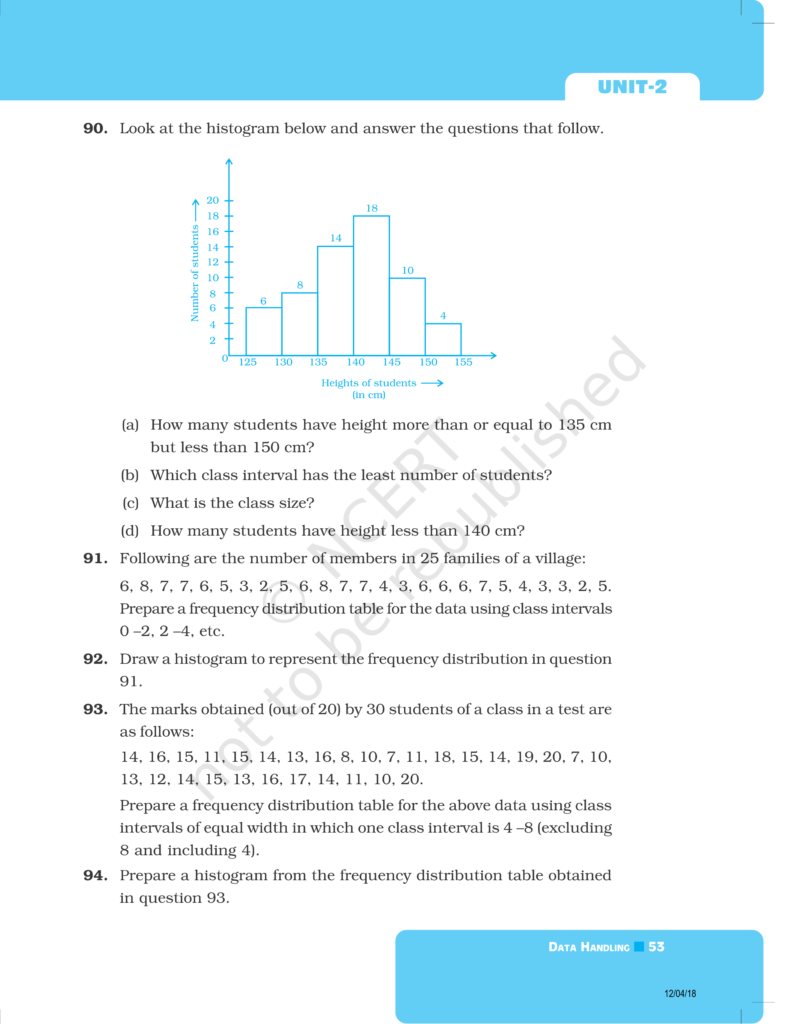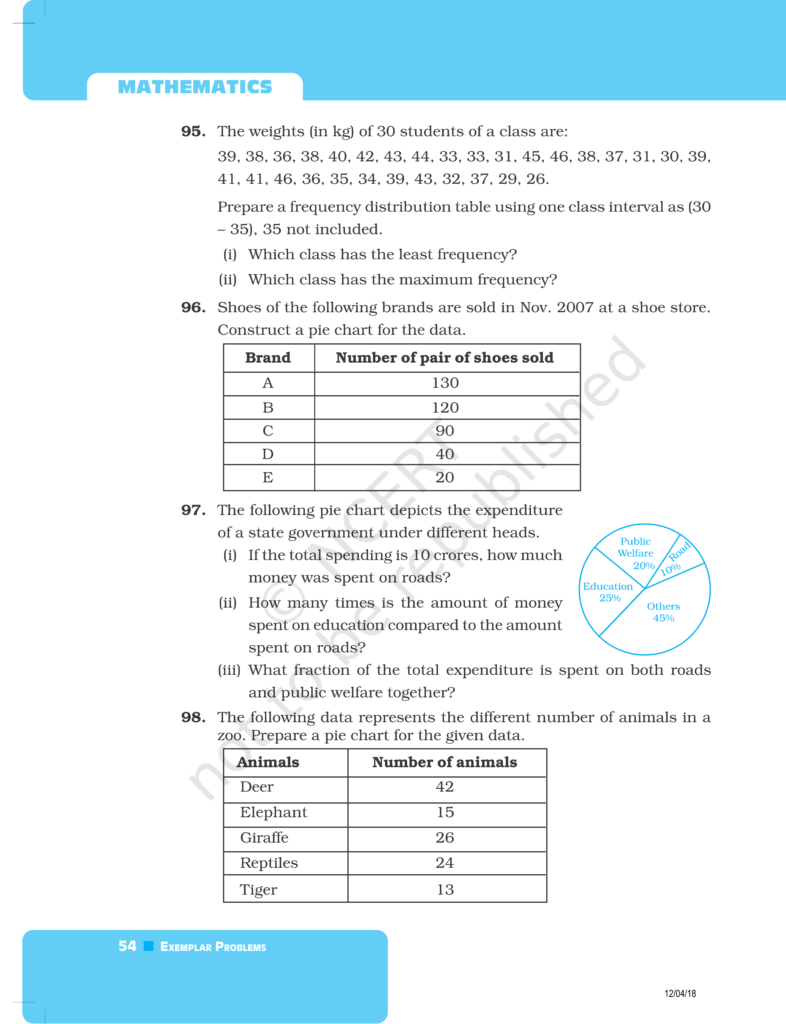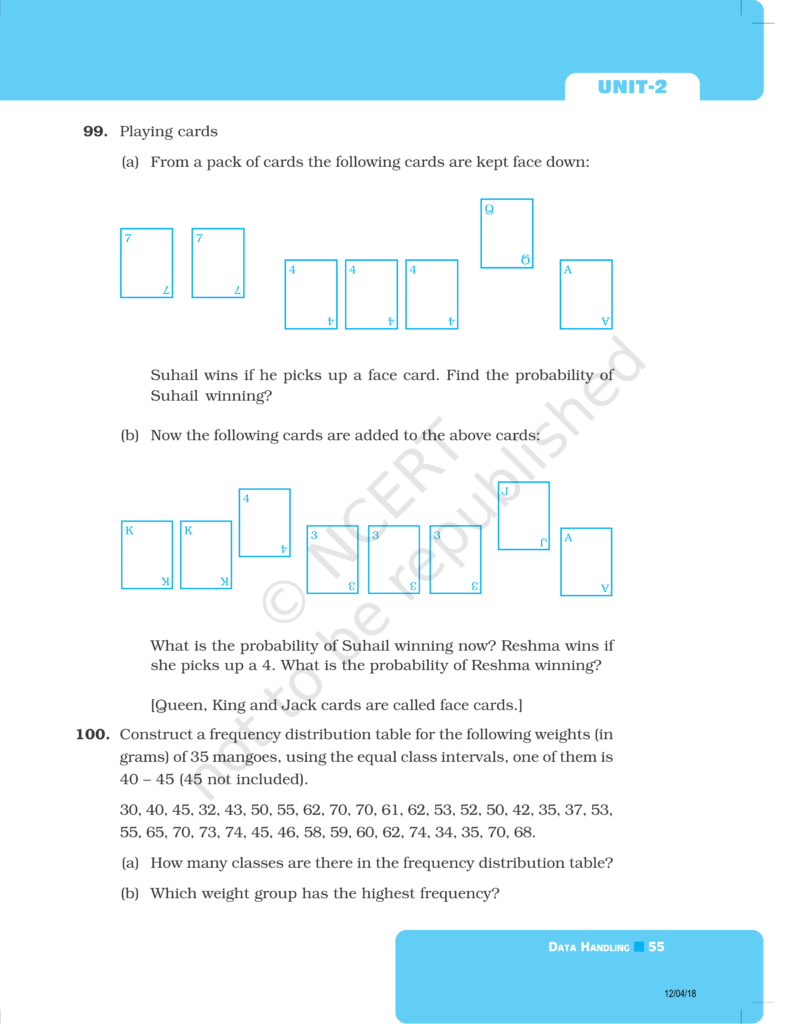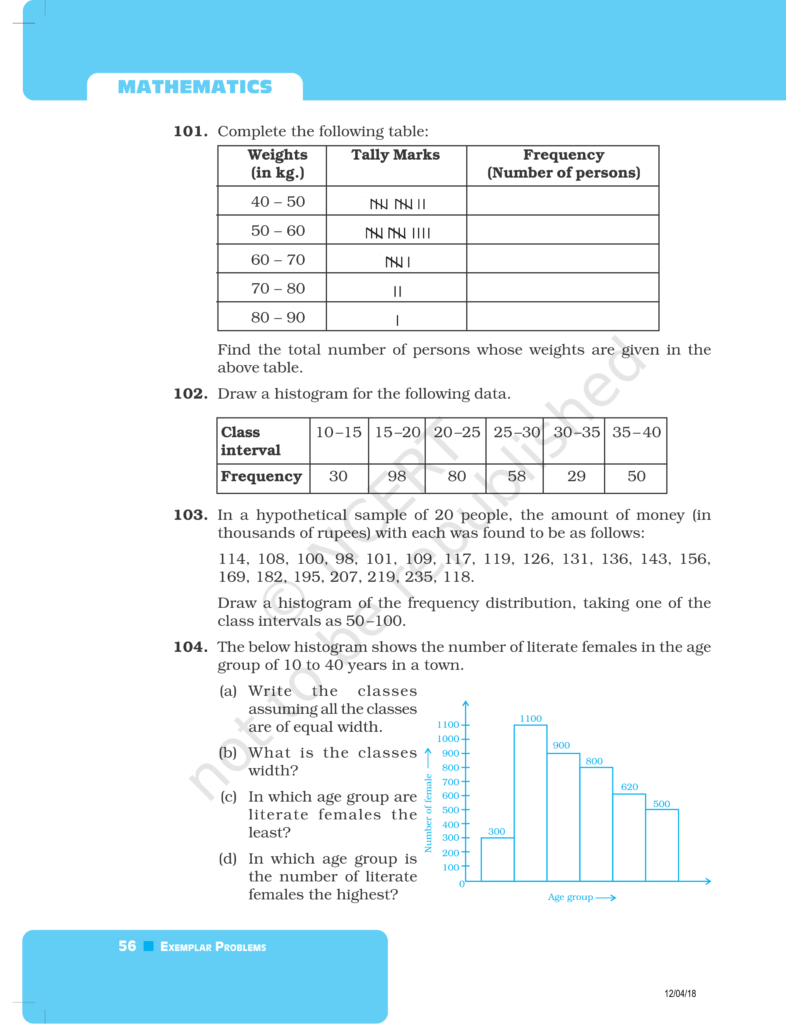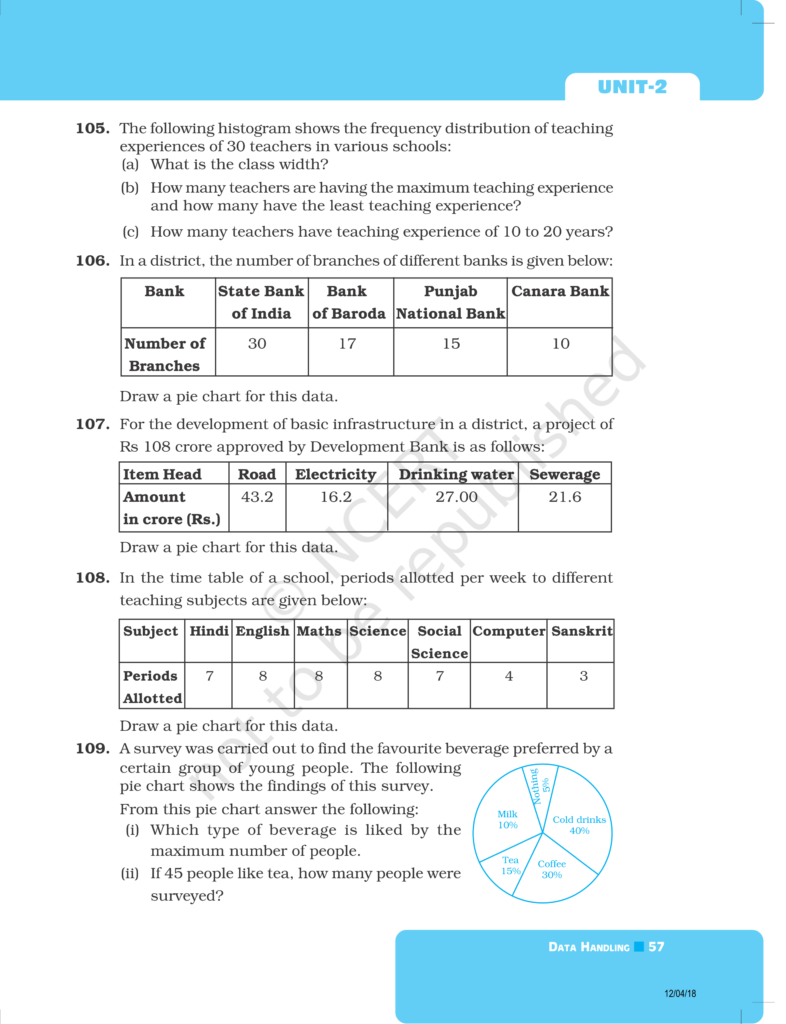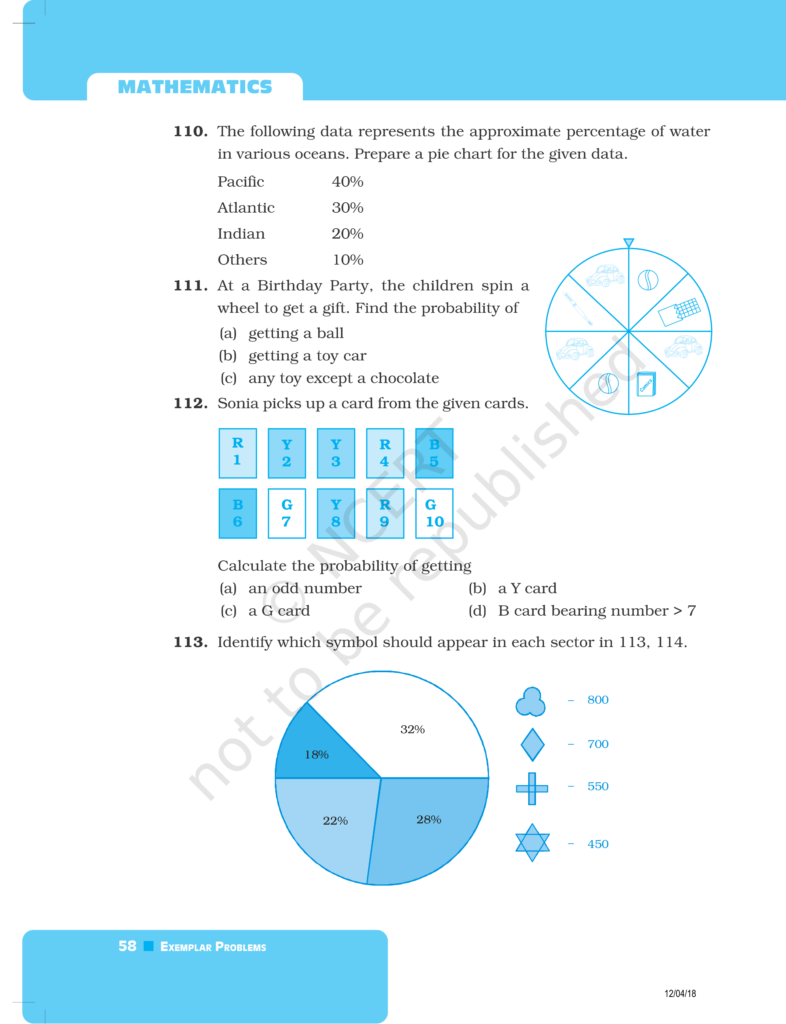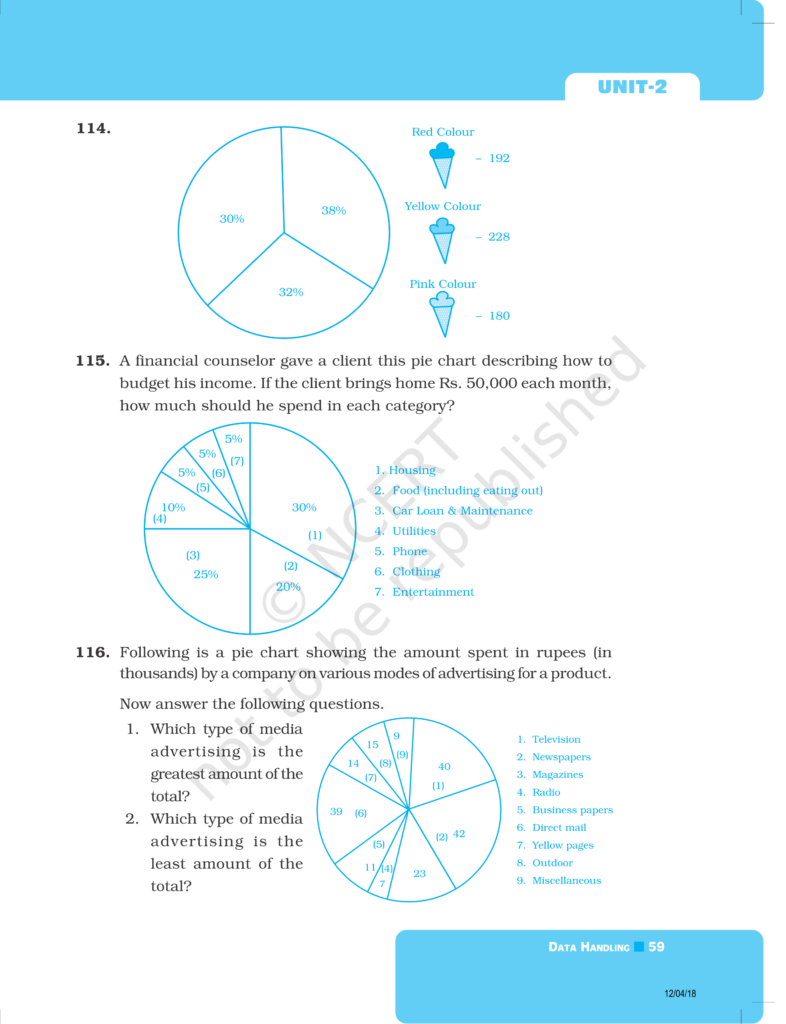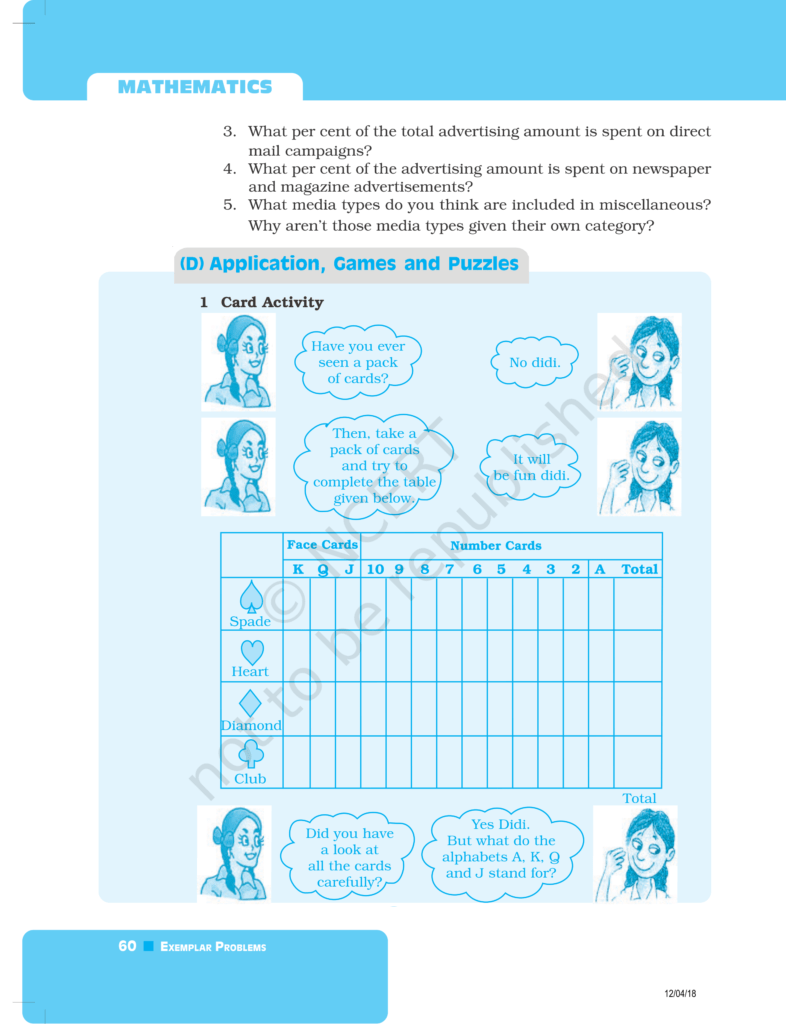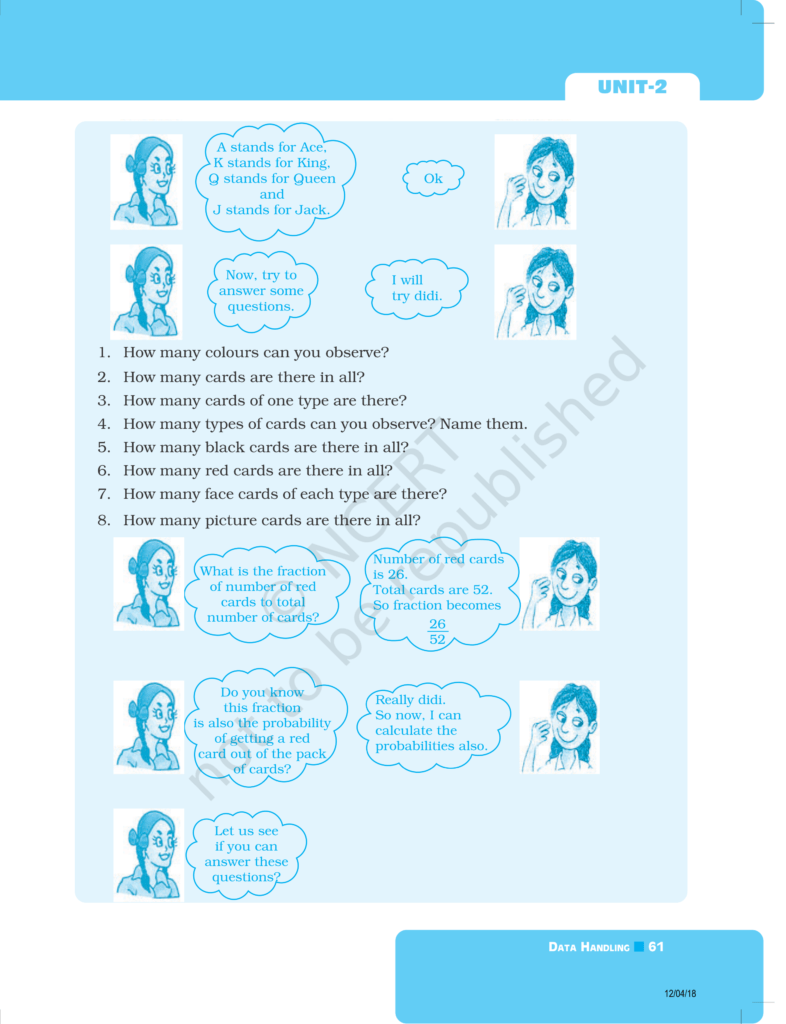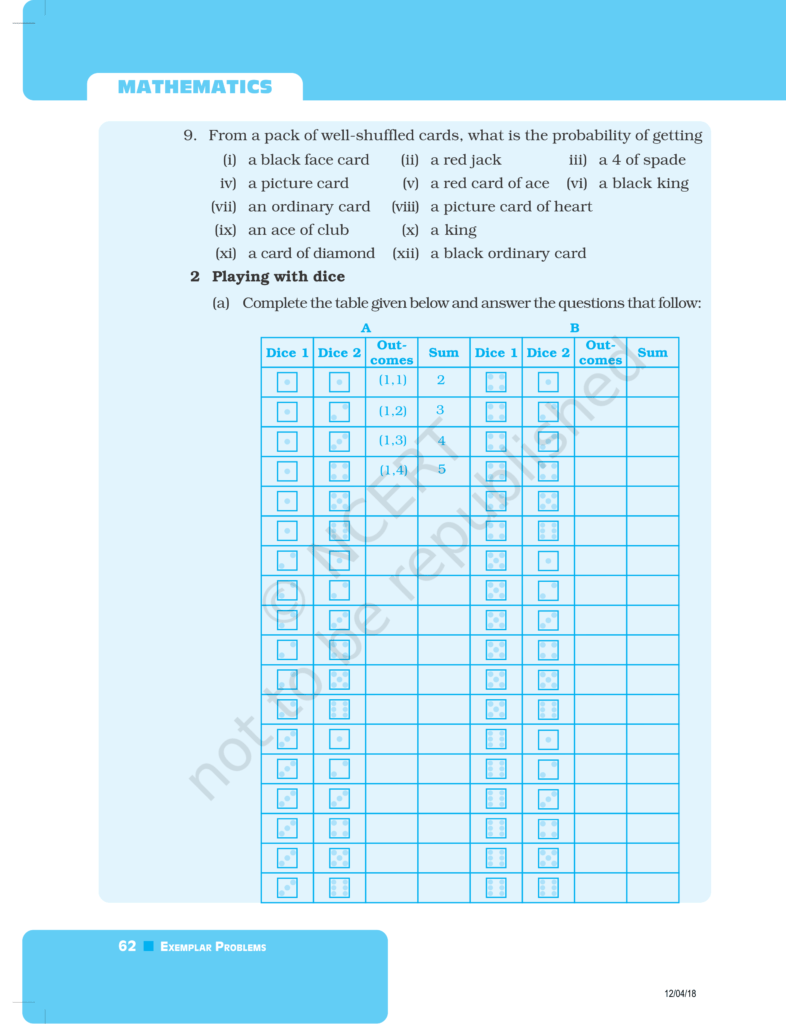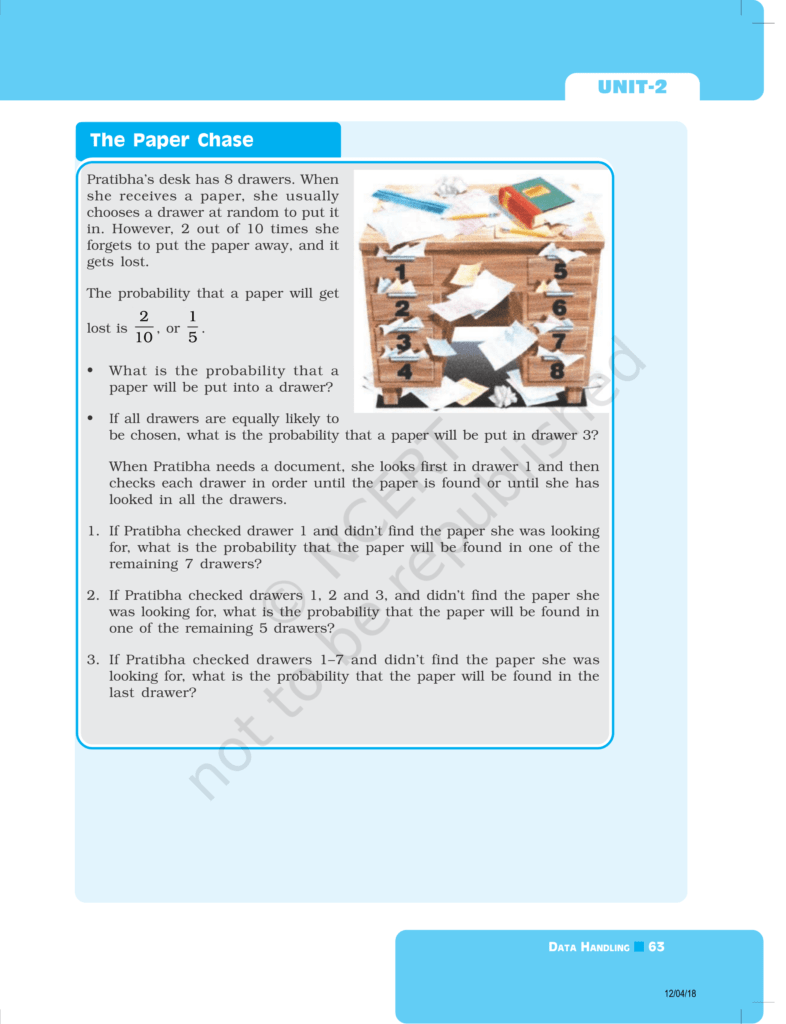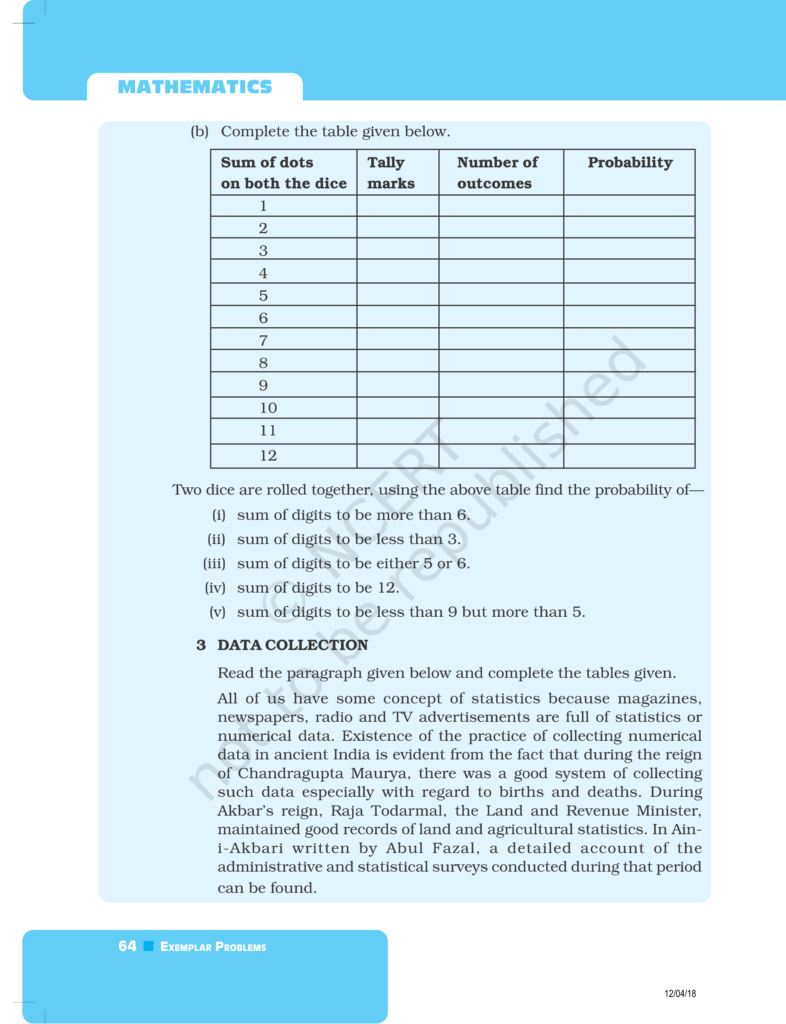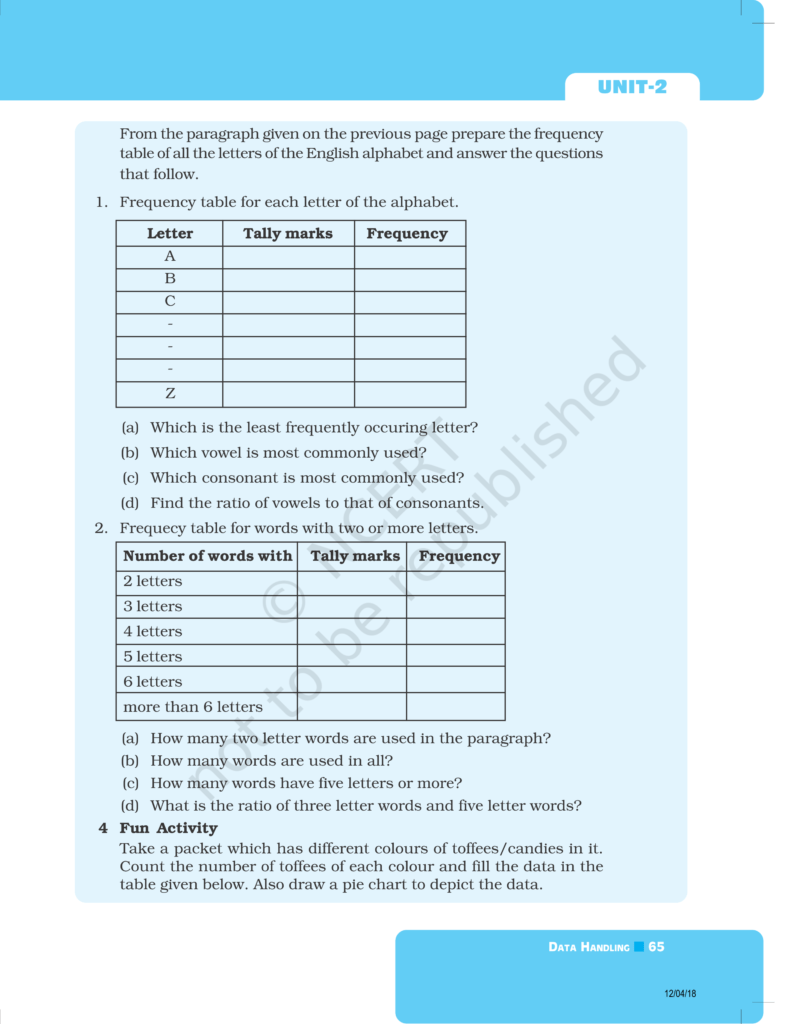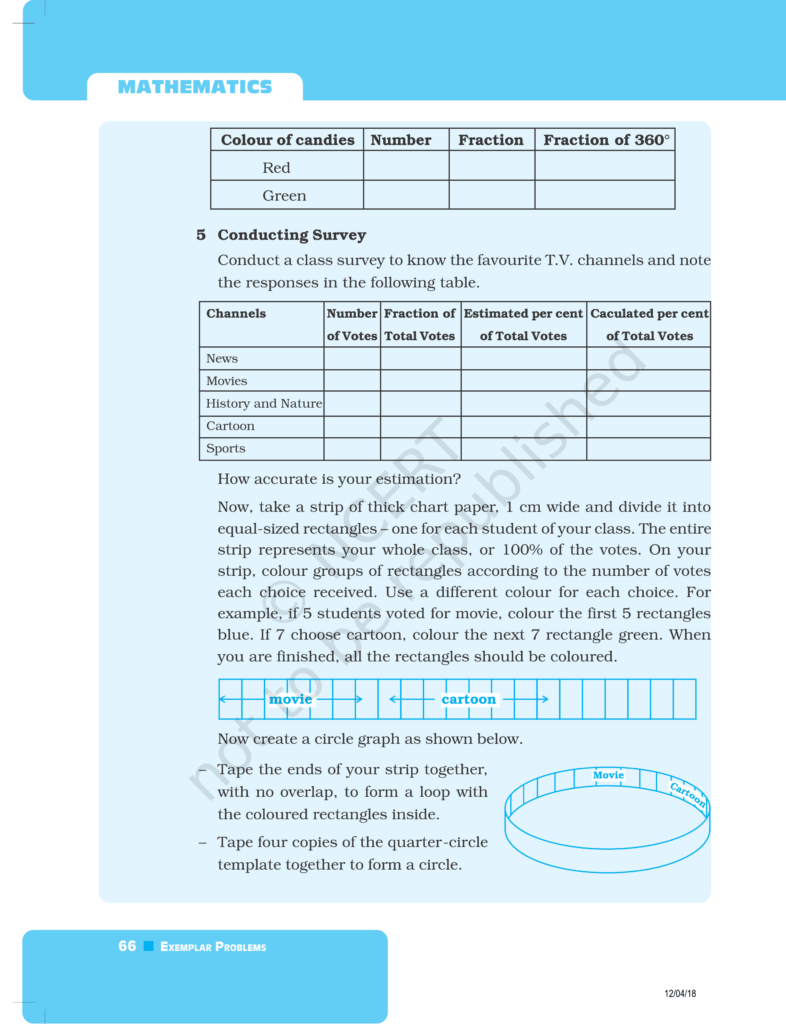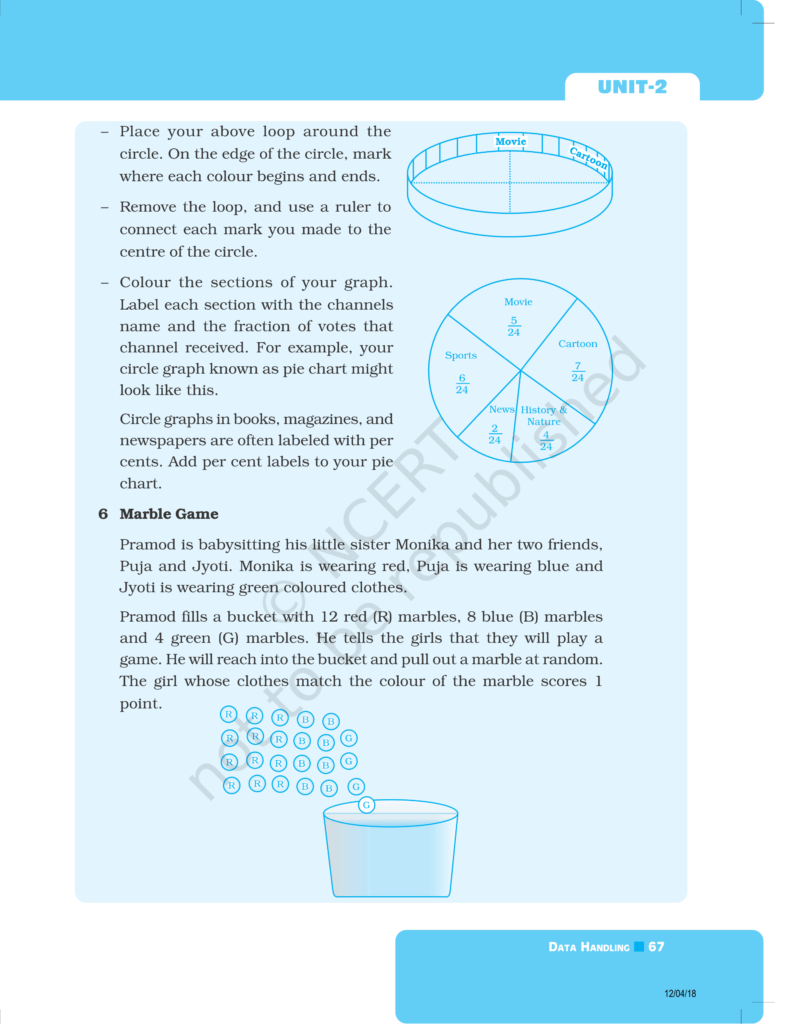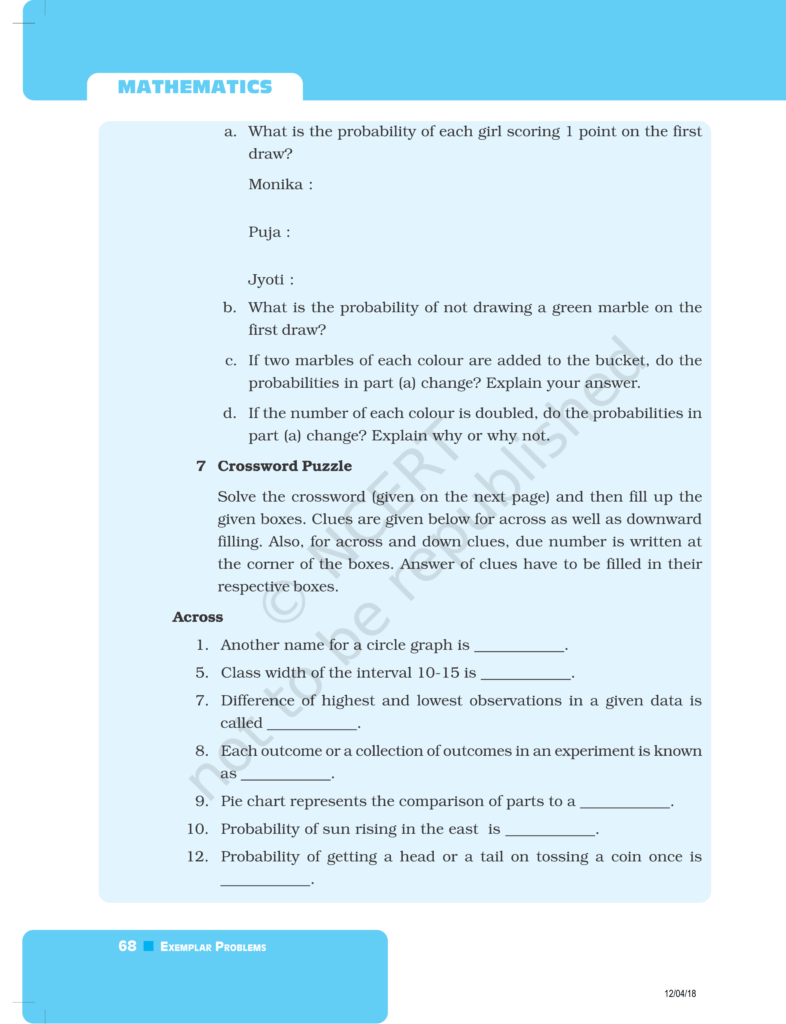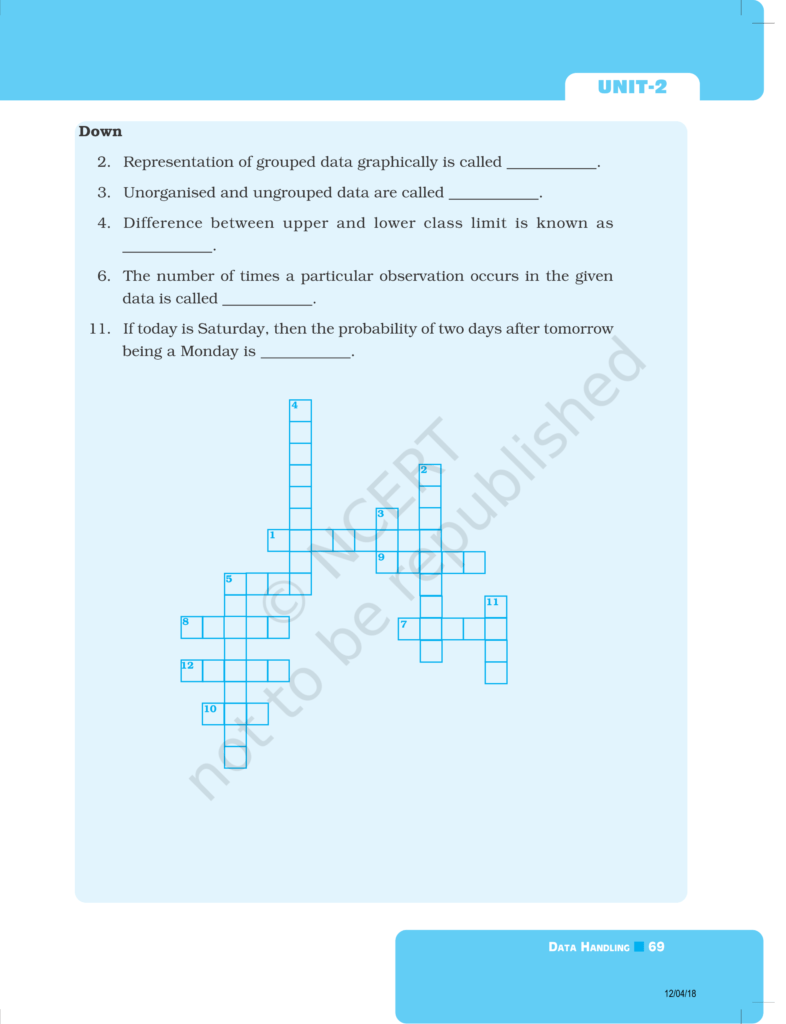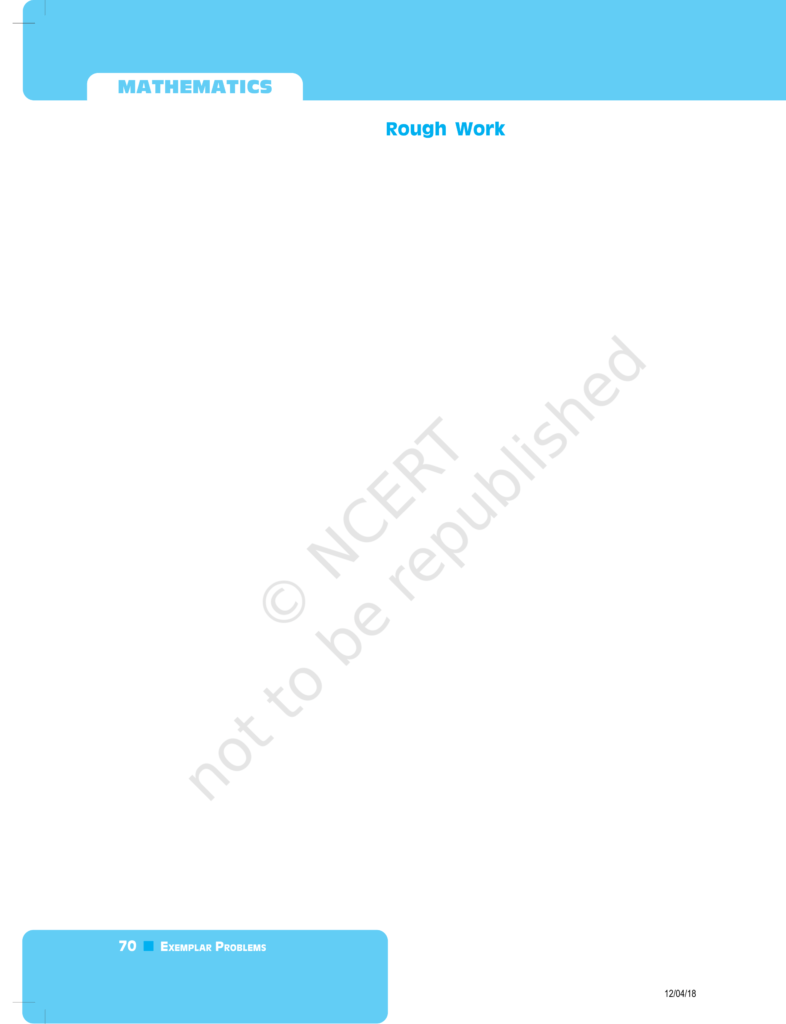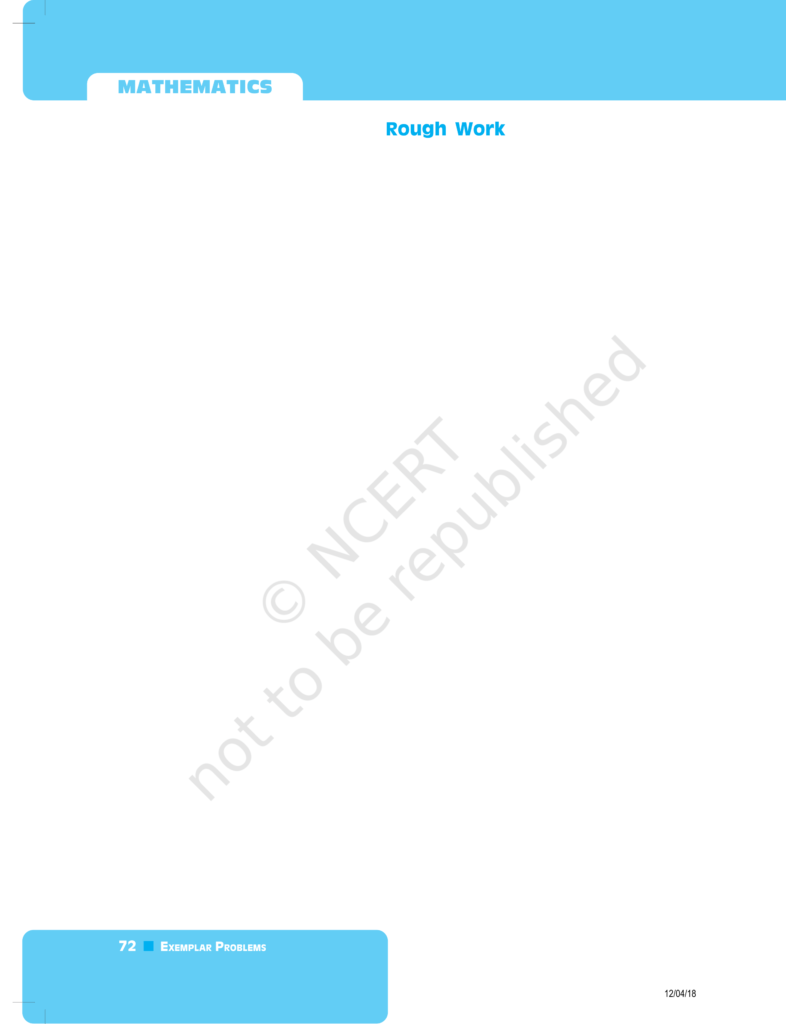NCERT Class 8 Maths Book PDF

NCERT Class 8 Science Book PDF

NCERT Exemplar Class 8 Science all chapters PDF

If you have any Confusion related to NCERT Exemplar Class 8 Maths Chapter 2 then feel free to ask in the comments section down below.

To watch Free Learning Videos on Class 8 Maths by Kota’s top Faculties Install the eSaral App